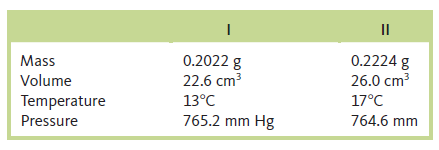# Problem: One of the chemical controversies of the nineteenth century concerned the element beryllium (Be). Berzelius originally claimed that beryllium was a trivalent element (forming Be3+ ions) and that it gave an oxide with the formula Be2O3. This resulted in a calculated atomic mass of 13.5 for beryllium. In formulating his periodic table, Mendeleev proposed that beryllium was divalent (forming Be2+ ions) and that it gave an oxide with the formula BeO. This assumption gives an atomic mass of 9.0. In 1894, A. Combes (Comptes Rendus 1894, p. 1221) reacted beryllium with the anion C5H7O2- and measured the density of the gaseous product. Combes’s data for two different experiments are as follows:If beryllium is a divalent metal, the molecular formula of the product will be Be(C 5H7O2)2; if it is trivalent, the formula will be Be(C5H7O2)3. Show how Combes’s data help to confirm that beryllium is a divalent metal.

###### FREE Expert Solution
85% (149 ratings)
###### Problem Details

One of the chemical controversies of the nineteenth century concerned the element beryllium (Be). Berzelius originally claimed that beryllium was a trivalent element (forming Be3+ ions) and that it gave an oxide with the formula Be2O3. This resulted in a calculated atomic mass of 13.5 for beryllium. In formulating his periodic table, Mendeleev proposed that beryllium was divalent (forming Be2+ ions) and that it gave an oxide with the formula BeO. This assumption gives an atomic mass of 9.0. In 1894, A. Combes (Comptes Rendus 1894, p. 1221) reacted beryllium with the anion C5H7O2- and measured the density of the gaseous product. Combes’s data for two different experiments are as follows:If beryllium is a divalent metal, the molecular formula of the product will be Be(C 5H7O2)2; if it is trivalent, the formula will be Be(C5H7O2)3. Show how Combes’s data help to confirm that beryllium is a divalent metal.

Frequently Asked Questions

What scientific concept do you need to know in order to solve this problem?

Our tutors have indicated that to solve this problem you will need to apply the The Ideal Gas Law: Density concept. You can view video lessons to learn The Ideal Gas Law: Density. Or if you need more The Ideal Gas Law: Density practice, you can also practice The Ideal Gas Law: Density practice problems.

What textbook is this problem found in?

Our data indicates that this problem or a close variation was asked in Chemistry: An Atoms First Approach - Zumdahl Atoms 1st 2nd Edition. You can also practice Chemistry: An Atoms First Approach - Zumdahl Atoms 1st 2nd Edition practice problems.